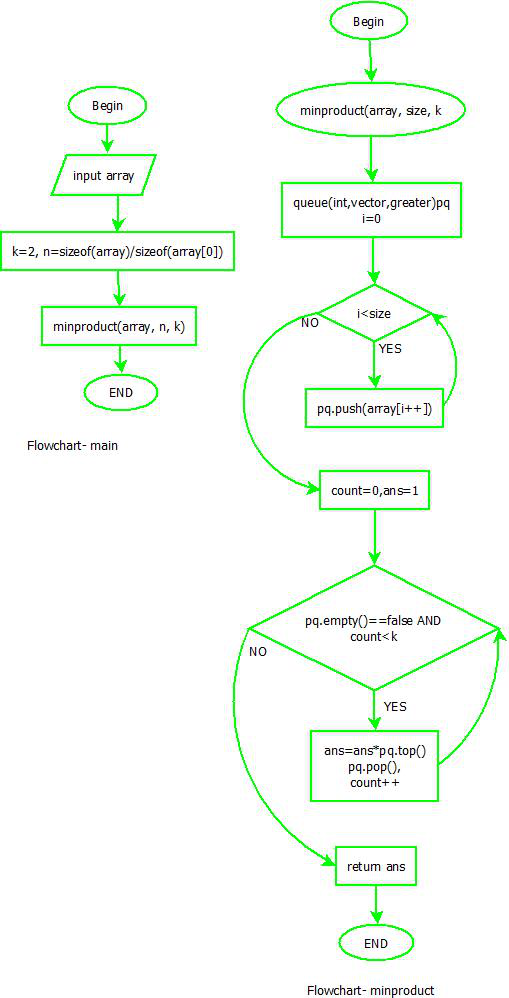# Minimum product of k integers in an array of positive Integers

• Difficulty Level : Easy
• Last Updated : 27 Jun, 2022

Given an array of n positive integers. We are required to write a program to print the minimum product of k integers of the given array.

Examples:

```Input : 198 76 544 123 154 675
k = 2
Output : 9348
We get minimum product after multiplying
76 and 123.

Input : 11 8 5 7 5 100
k = 4
Output : 1400```

The idea is simple, we find the smallest k elements and print multiplication of them. In the below implementation, we have used a simple Heap-based approach where we insert array elements into a min-heap and then find product of top k elements.

Flowchart:Flowchart

## C++

 `// CPP program to find minimum product of` `// k elements in an array` `#include ` `using` `namespace` `std;`   `int` `minProduct(``int` `arr[], ``int` `n, ``int` `k)` `{` `    ``priority_queue<``int``, vector<``int``>, greater<``int``> > pq;` `    ``for` `(``int` `i = 0; i < n; i++)` `        ``pq.push(arr[i]);`   `    ``int` `count = 0, ans = 1;`   `    ``// One by one extract items from max heap` `    ``while` `(pq.empty() == ``false` `&& count < k) {` `        ``ans = ans * pq.top();` `        ``pq.pop();` `        ``count++;` `    ``}`   `    ``return` `ans;` `}`   `// Driver code` `int` `main()` `{` `    ``int` `arr[] = { 198, 76, 544, 123, 154, 675 };` `    ``int` `k = 2;` `    ``int` `n = ``sizeof``(arr) / ``sizeof``(arr);` `    ``cout << ``"Minimum product is "` `<< minProduct(arr, n, k);` `    ``return` `0;` `}`

## Java

 `// Java program to find minimum product of ` `// k elements in an array`   `import` `java.util.PriorityQueue;`   `class` `GFG` `{` `    ``public` `static` `int` `minProduct(``int``[] arr, ``int` `n, ``int` `k) ` `    ``{` `        ``PriorityQueue pq = ``new` `PriorityQueue<>();` `        ``for` `(``int` `i = ``0``; i < n; i++)` `            ``pq.add(arr[i]);` `        `  `            ``int` `count = ``0``, ans = ``1``;`   `            ``// One by one extract items` `            ``while``(pq.isEmpty() == ``false` `&& count < k)` `            ``{` `                ``ans = ans * pq.element();` `                ``pq.remove();` `                ``count++;` `            ``}` `        `  `            ``return` `ans;` `    ``}`   `    ``// Driver Code` `    ``public` `static` `void` `main(String[] args)` `    ``{` `        ``int` `arr[] = {``198``, ``76``, ``544``, ``123``, ``154``, ``675``};` `        ``int` `k = ``2``;` `        ``int` `n = arr.length;` `        ``System.out.print(``"Minimum product is "` `+ ` `                          ``minProduct(arr, n, k));` `    ``}` `}`   `// This code is contributed by sanjeev2552`

## Python3

 `# Python3 program to find minimum` `# product of k elements in an array`   `import` `math ` `import` `heapq`   `def` `minProduct(arr, n, k):`   `    ``heapq.heapify(arr)` `    ``count ``=` `0` `    ``ans ``=` `1`   `    ``# One by one extract ` `    ``# items from min heap` `    ``while` `( arr ) ``and` `count < k:` `        ``x ``=` `heapq.heappop(arr)` `        ``ans ``=` `ans ``*` `x` `        ``count ``=` `count ``+` `1` `    `  `    ``return` `ans;`   `# Driver method` `arr ``=` `[``198``, ``76``, ``544``, ``123``, ``154``, ``675``]` `k ``=` `2` `n ``=` `len``(arr)` `print` `(``"Minimum product is"``,` `       ``minProduct(arr, n, k))`

## C#

 `// C# program to find minimum product of ` `// k elements in an array`   `using` `System;` `using` `System.Collections.Generic;` `public` `class` `GFG` `{` `  ``public` `static` `int` `minProduct(``int``[] arr, ``int` `n, ``int` `k) ` `  ``{` `    ``List<``int``> pq = ``new` `List<``int``>();` `    ``for` `(``int` `i = 0; i < n; i++)` `      ``pq.Add(arr[i]);`   `    ``int` `count = 0, ans = 1;`   `    ``// One by one extract items` `    ``while``(pq.Count!=0 && count < k)` `    ``{` `      ``pq.Sort();` `      ``ans = ans * pq;` `      ``pq.RemoveAt(0);` `      ``count++;` `    ``}`   `    ``return` `ans;` `  ``}`   `  ``// Driver Code` `  ``public` `static` `void` `Main(String[] args)` `  ``{` `    ``int` `[]arr = {198, 76, 544, 123, 154, 675};` `    ``int` `k = 2;` `    ``int` `n = arr.Length;` `    ``Console.Write(``"Minimum product is "` `+ ` `                  ``minProduct(arr, n, k));` `  ``}` `}`   `// This code is contributed by Rajput-Ji `

## Javascript

 ``

Output

`Minimum product is 9348`

Time Complexity: O(n * log n), Auxiliary Space: O(n) for priority queue

Note: The above problem can be solved in O(n) time using methods discussed here and here.

This article is contributed by Gitanjali Sharma. If you like GeeksforGeeks and would like to contribute, you can also write an article using write.geeksforgeeks.org or mail your article to review-team@geeksforgeeks.org. See your article appearing on the GeeksforGeeks main page and help other Geeks.